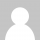# for 循环为何可恨？

Java的闭包(Closure)特征最近成为了一个热门话题。一些精英正在起草一份议案，要在Java将来的版本中加入闭包特征。然而，提议中的闭包语法以及语言上的这种扩充受到了众多Java程序员的猛烈抨击。

```3.times {puts "Inside the times method."}

Inside the times method.
Inside the times method.
Inside the times method.```

`times`是3这个对象上的一个方法。它把闭包中的代码执行了3次。`{puts "Inside the times method."}`是闭包。它是一个匿名函数，把它传入times方法，打印出静态句子。相比起传统的for循环语句，这样的代码显得更紧凑，更简单，如表2中所示：

```for i in 1..3
puts "Inside the times method."
end```

```double sum = 0;
for (int i = 0; i < array.length; i++) {
sum += array[i];
}```

```String s = "";
for (int i = 0; i < args.length; i++) {
s += array[i];
}```

`total = sum array`

`total = foldl (+) 0 array`

```s = concat array
s = foldr (++) [] array```

```s = foldl (+) 0       [1, 2, 3]
= foldl (+) (0 + 1) [2, 3]
= foldl (+) 1       [2, 3]
= foldl (+) (1 + 2) 
= foldl (+) 3       
= foldl (+) (3 + 3) []
= foldl (+) 6       []
= 6```

Haskell语言里提供了很多fold函数；`foldl`函数从list的第一位开始运算，依次反复到最后一个，而`foldr`函数，它从list的最后一个函数开始运算，从后往前。还有很多其它相似的函数，但这两个是最基本的。

```for (int i = 0; i < array.length; i++) {
array[i] *= 2;
}```

`new_array = map (*2) array`

`map`函数的工作方式是，它会检查list里的每个元素，将一个函数应用到每个元素上，形成一个新的list，里面是新的元素。（有些语言里的这种操作是原位替换）。这是一个很容易理解的操作。`sort`函数的功能相似，它接受一个list，返回(或修改)一个list。

```int tmp[] = new int[nums.length];
int j = 0;
for (int i = 0; i < nums.length; i++) {
if ((nums[i] % 2) == 1) {
tmp[j] = nums[i];
j++;
}
}```

```odds = filter (\i => (i `mod` 2) == 1) nums
odds = filter isOdd nums -- 更常用的形式```

### 16 Responses to for 循环为何可恨？

1.ammo says:

妙哉我大Linq

2.haitao says:

c的for只是提供了：初始条件，循环中止判断规则，循环递增方式
是非常灵活的。
如果只是加1递增，是可以提供一个更简单的：那就是pascal的for（它也可以减递减）。

•champ says:

确实，如果算法中for的终止条件和递增方式都与步骤中间结果有关，就无法简单的用map/reduce/filter去处理。

•dohkoos says:

没有万能的解决方案，各有各的利弊，关键在于如何找到平衡。for循环代码中符号众多，容易出现bug，可是它灵活。

3.paladin_t says:

“做我们想要的事，比如sum and prod”是否应作“做我们想要的事，比如sum和prod”？
文章不错。

•Aqee says:

谢谢提醒，已改正

4.stopit says:

拜读了~

5.liango says:

这四行代码里大概散布着30多个标记符号需要我去分析处理

•liango says:

30多个在哪儿？

•依云 says:

没数错的话是 31 个 tokens。

6.lite3 says:

我感觉这更像设计模式里提到的那样的,将变化的和不变化的分别独立出来,不要将它们混在一起.

7.flyfish30 says:

下面这句话翻译的不大好
如果你把一个可能进行并行运算的操作(例如map 和 filter)分解成连续的运算(例如foldl 和 foldr)，编译器更容易从中做出判断。
翻译成下面这样要好些
如果你能将串行计算（即foldl和foldr）从可能并行化的计算（即map和filter）中分离出来，这将比由编译器来分离这些计算更容易得多。

这一段是针对现在编译器在并行优化上的一些缺点来说的。在只有for循环的loop中，编译器是很难对复杂的循环遍历和计算操作作出很好的并行优化的。如果我们采用函数式的思维习惯来设计程序，将我们的复杂计算表述为fold、map、filter、combination的形式，编译器将可以很好的进行并行优化。当你对自己的数据结构的理解比编译器更好时，甚至可以显式的指明采用并行版或串行版的map。

•aqee says:

it’s much easier for the compiler to figure out
你把这句理解成“这将比由编译器来分离这些计算更容易得多”，感觉有些不妥，你觉得呢？

8.o0o0o says:

这些函数不就是对for循环的包装吗？内部难道不是用for循环，反正都要遍历。喜欢这样的函数，可以自己写啊。
int Sum（int[] array）
{
double sum = 0;
for (int i = 0; i < array.length; i++) {
sum += array[i];
}
}

•o0o0o says:

double Sum（double[] array）
{
double sum = 0;
for (int i = 0; i < array.length; i++) {
sum += array[i];
}
return sum;
}

9.Yangff says:

for(qsort(a,s,8,cmp);s–;b[ra[a[s]]=ra[a[s+1]]+(a[s]!=a[s+1])]=a[s]);
快用each\filter来写。